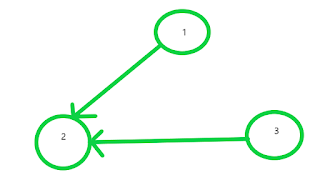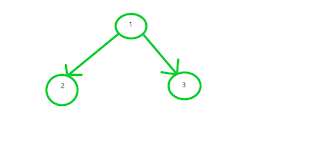# Determine if every node of tree is accessible from any one node of the tree by performing atmost N/2 operations

#### Problem Statement

Given an directed Tree,determine whether there exists one node of the tree from where all other nodes are accessible by performing following operations not more than (floor) n/2 times.

Operations are defined as:

• Choose some directed edge of the graph and remove it
• Add another directed edge between any two nodes in the graph

You have to determine whether it is possible or not.Output->true/false

Example 1:

In the given example we can remove edge between node 3  and node 2

and add an edge between node 1 and node 3.Total operations=1Before OperationAfter Operation

Output:true

Example 2:Input image

Output:false

Solution:One important observation which we can draw is that every node should have atleast one parent i.e in other words every node should have atleast 1 indegree to make tree accessible,but this does not holds true only for the node from which all other nodes will be accessible.So,if in a tree every node atleast has one indegree(except the node from which all other nodes will be accessible)than it is possible to access all nodes in that tree.This brings the conclusion that if we can make every node atleast 1 indegree than we can access all other nodes.Our task got reduced to count nodes which have NO indegree and check if it is ≤ n/2.

Below is the implementation of the Above approach:

import java.io.*;
import java.util.HashMap;

class Solution {
public static void main(String[] args)
{
int n=3;
HashMap<Integer,Integer> map=new HashMap<>();
//creating a map to store
//number of parent of each node
map.put(1,0);
map.put(2,2);
map.put(3,0);
//calling function
System.out.println(find(map,n));
}
public static boolean find(HashMap<Integer,Integer> map,int n)
{
int[] a = new int[n];
for (int i = 0; i < n; i++) {
a[i] = map.getOrDefault(i + 1, 0);
}
int count0 = 0;
for (int i = 0; i < n; i++) {
// if the ith node doesnt
// have any parent then
// increasing operation count
if (a[i] == 0)
{
count0++;
}
}
count0 -= 1;
//if number of operations
//needed is less than floor
// (n/2) then output true
//else output false
if(count0<=Math.floor(((double) n)/((double) 2)))
{
return true;
}
return false;
}
}

Time Complexity:O(n)

Feel free to comment if you have any doubt in comment section

For more Data structure and algorithms,computer science,programming,coding related problems search my website.

Learn how to prepare for Information Technology Based companies here